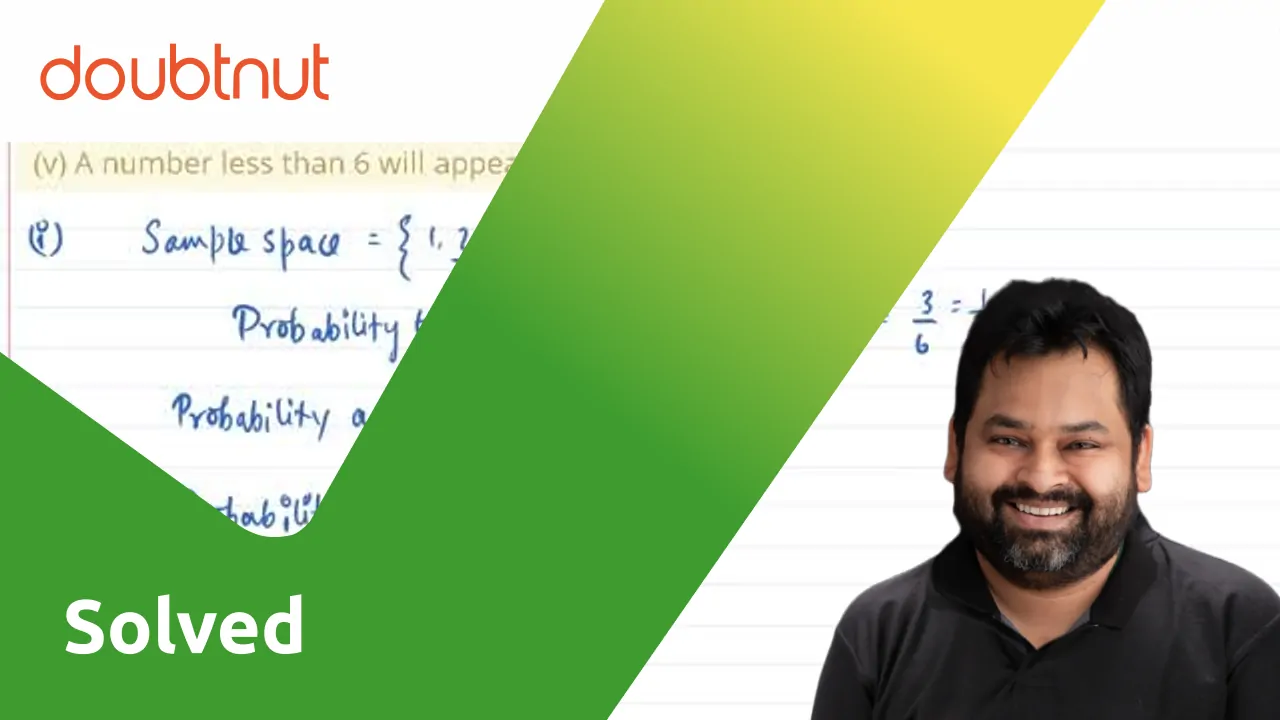Doubtnut App - Get 50 Lakh+ video solutions better than GoogleInstall Now

# A die is thrown, find the probability of following events: (i) A prime number will appear, (ii) A number greater than or equal to 3 will appear, (iii) A number less than or equal to one will appear, (iv) A number more than 6 will appear, (v) A number less than 6 will appear.

Text Solution
Verified by Experts

## The sample space of the given experiment is given by S={1,2,3,4,5,6} (i) Let A be the event of the occurrence of a prime number. Accordingly, A={2,3,5} P(A)=36=12 (ii) Let B be the event of the occurrence of a number greater than or equal to 3. Accordingly, B = {3, 4, 5, 6} P(B)=46=23 (iii) Let C be the event of the occurrence of a number less than or equal to one. Accordingly, C = {1} P(C)=16 (iv) Let D be the event of the occurrence of a number greater than 6. Accordingly, D=ϕ P(D)=06=0 (v) Let E be the event of the occurrence of a number less than 6. Accordingly, E = {1, 2, 3, 4, 5} P(E)=56